# Test: Identify Class Language

## 10 Questions MCQ Test GATE Computer Science Engineering(CSE) 2023 Mock Test Series | Test: Identify Class Language

Description
Attempt Test: Identify Class Language | 10 questions in 20 minutes | Mock test for Computer Science Engineering (CSE) preparation | Free important questions MCQ to study GATE Computer Science Engineering(CSE) 2023 Mock Test Series for Computer Science Engineering (CSE) Exam | Download free PDF with solutions
QUESTION: 1

Solution:

L is regular.

QUESTION: 2

### If L1 is context free language and L2 is a regular language which of the following is/are false? a) L1-L2 is not context free b) L1 ∩ L2 is context free c) ~L1 is context free d) ~L2 is regular

Solution:
• Given L1 is a context free language and L2 as a regular language then L1-L2 is context free language and
• ~L1(complement of context free) is not context free because context free is not closed under complement.
• Complement of regular language i.e. ~L2 is also regular ( so d is correct ) since statements a and c are false.

Hence,Option (C) is correct.

QUESTION: 3

### Let  denote the languages generated by the grammar S→ 0S0 I 00. Which of the following is TRUE?

Solution:

Language generated by this grammar is L = (00)^+ that is regular but not 0^+

QUESTION: 4

The language accepted by a Pushdown Automaton in which the stack is limited to 10 items is best described as

Solution:

With only finite positions in stack, we can have only finite configurations and these can also be modeled as states in a finite
automata.

QUESTION: 5

The language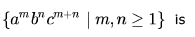Solution:

Language is not regular bcoz we need to match count of c's is equal to count of b's + count of a's

and that can implement by PDA

∂(q0,a,^)= (q0,a) [ push a in stack, as per language a comes first]

∂(q0,a,a)= (q0,aa) [push all a's into stack]
∂(q0,b,a) = (q1,ba) [push b in stack, state change to q1 that sure b comes after a]
∂(q1,b,b)=(q1,bb) [push all b's in stack]
∂(q1,c,b) = (q2,^) [ pop one b for one c]

∂(q2,c,b) = (q2,c) [ pop one b's for each c and continue same ]
∂(q2,c,a) = (q3,^) [ pop one a for one c , when there is no more b in stack]
∂(q3,c,a) = (q3,^) [pop one a for each c and continue same]
∂(q3,^,^) = (qf,^) [ if sum of c's is sum of a's and b's then stack will be empty when there is no c in input]

Note :1. state changes make sure b's comes after a and c's comes after b's]

QUESTION: 6

Consider the languages: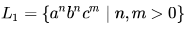and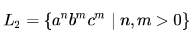Which one of the following statements is FALSE?

Solution:

L1 is CFL [ put a's in stack, and pop a with each b]]
L2 is CFL [put b's in stack and pop b with each c ]
c) is True.

b) is True CFL is closed under Union [ S-> S1 | S2 where S1 is grammar for L1 and S2 for L2]
CFL is not closed under Intersection, so intersection of two CFLs may or may not be CFL.

Let's examine:
L1 ∩ L2 = { aibici , i>0 } which is Context sensitive but not context free [can't match a's,b's and c's with one stack]
So a) is False

d) is True

QUESTION: 7

Let L be a regular language and M be a context-free language, both over the alphabet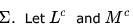denote the complements of  L and M respectively. Which of the following statements about the language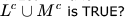Solution:

Take L = Σ* then Lc = ∅ and Mc ∪ ∅ = Mc.

We know that complement of CFL need not be a CFL as CFL is not closed under complement

So, (A) and (B) are false.

If we take L = ∅ then Lc = Σ* and Mc ∪ Σ* = Σ* which is regular - (C) is also false.

QUESTION: 8

The language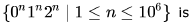Solution:

Regular (in fact finite). Since n is finite, we have a finite set of strings satisfying the given conditions.

So, we can easily make a finite automata for those strings.

QUESTION: 9

For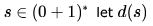denote the decimal value of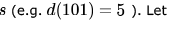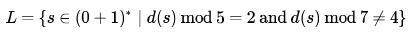Which one of the following statements is true?

Solution:

L1 = { s∈(0+1)* ∣ d(s) mod 5=2 } is regular having 2 as final state out of {0,1,2,3,4} states as given in example in posted link [in same DFA , final state will be 2 instead of 0 ]

Similarly L2 = { s∈(0+1)* ∣ d(s) mod 7≠4 } is also regular having states {0,1,2,3,4,5,6} and all are final state except 4
L1 ∩ L2 is L (given problem ) is also regular
As regular languages are closed under intersection.

Hence, D is the correct option.

QUESTION: 10

Let L1 be a regular language L2,  be a deterministic context-free language and L3 a  recursively enumerable, but not recursive, language. Which one of the following statements is false?

Solution:

A. True: DCFL are closed under Intersection with Regular Languages
C. True: L1 is regular hence also CFL and every DCFL is also CFL and All CFL are closed under Union.
D. True: L1 is regular hence also RE; L2 is DCFL hence also RE; RE languages are closed under Intersection
B. False: L1 is recursive hence also decidable, L3 is RE but not Recursive hence it is undecidable. Intersection of Recursive
language and Recursive Enumerable language is Recursive Enumerable language.Use Code STAYHOME200 and get INR 200 additional OFF Use Coupon Code